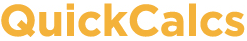1. Select category 2. Choose calculator 3. Enter data 4. View results

## Sign and binomial test

 Use the binomial test when there are two possible outcomes. You know how many of each kind of outcome (traditionally called "success" and "failure") occurred in your experiment. You also have a hypothesis for what the true overall probability of "success" is. The binomial test answers this question: If the true probability of "success" is what your theory predicts, then how likely is it to find results that deviate as far, or further, from the prediction. The sign test is a special case of the binomial case where your theory is that the two outcomes have equal probabilities.    Number of "successes" you observed =    Number of trials or experiments = You will compare those observed results to hypothetical results. What is the hypothetical probability of "success" in each trial or subject? (For a sign test, enter 0.5.)    Probability =